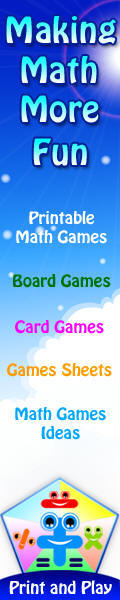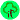# Interactive Stuff: at the Interactivities Forum

###### Teachers and stdents, creating multimedia in class# Mathematical Matching Puzzles

### Featured Puzzles

3D Shapes 1 Which 3D solid is which?

Abacus Which abacus shows which number?

Angles 1 Find the angles which match the diagrams on the cards

Bearings Match each bearing with the diagram which represents the direction you would face if you turned that way.

Coloured Shapes 1 Match each shape and colour with its correct description

Division 1 Testing multiplication table facts by finding the answers to division questions

Division 2 Match the different division questions to their answers

Division 3 Testing multiplication table facts by finding the answers to division questions

Dots Match the number of dots to the correct words that describe them in this simple puzzle.

Fractions 1 Can you convert fractions to decimals?

Fractions 2 A mixture of fractions, percentages and decimals. Which pairs are the same?

Mixed Multiplication 1 Testing multiplication table facts by finding the answers to multiplication questions

Multiplication 2 Testing multiplication table facts by finding the multiplication questions which have the same answersSets of Numbers Match each set of numbers with its name - but to make it more interesting, you must also identify the odd one out for each collection of four numbers by clicking on it.Shape Match 1 Click on the correct coloured shapes to match their names.Shape Match 2 Click on the correct coloured shapes to match their names.